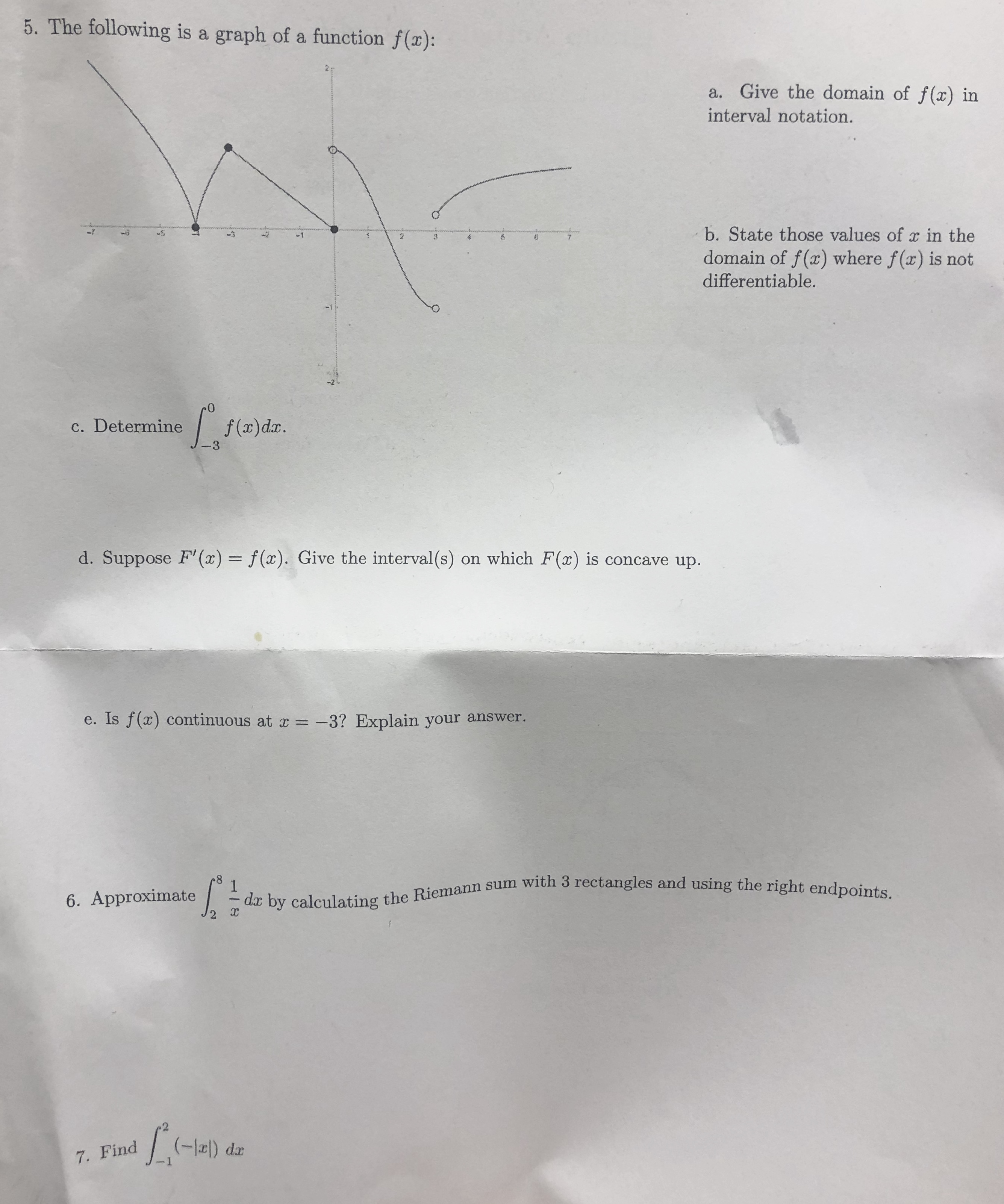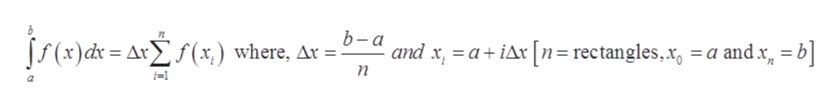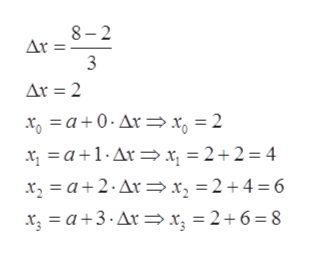# 5. The following is a graph of a function f(x):a. Give the domain of f(x) ininterval notation.b. State those values of r in the-337domain of f(x) where f(x) is notdifferentiable.efa)da.c. Determine-3d. Suppose F' (x) = f(x). Give the interval(s) on which F(x) is concave up.e. Is f(x) continuous at x-3? Explain your answer.- da by calculating the Riemann sum with 3 rectangles and using the right endpoints.16. Approximate7. Find

Question
1 views

Question 6help_outlineImage Transcriptionclose5. The following is a graph of a function f(x): a. Give the domain of f(x) in interval notation. b. State those values of r in the -3 3 7 domain of f(x) where f(x) is not differentiable. efa)da. c. Determine -3 d. Suppose F' (x) = f(x). Give the interval(s) on which F(x) is concave up. e. Is f(x) continuous at x -3? Explain your answer. - da by calculating the Riemann sum with 3 rectangles and using the right endpoints. 1 6. Approximate 7. Find fullscreen
check_circle

Step 1

Given, function is

Step 2

Reimann right sum is given byhelp_outlineImage Transcriptioncloseb-a ff (s) ά= ΔΣ (x) where, Δr and x, aiAr n=rectangles,x, =a and x b п fullscreen
Step 3

So, the value of...help_outlineImage Transcriptionclose8-2 Ar = 3 Ar 2 xo a0.Ar x, = 2 a1Ar x, = 2+2 = 4 x, = 2 + 4 = 6 xa2.Ar x a+3. Arx, = 2 + 6 = 8 fullscreen

### Want to see the full answer?

See Solution

#### Want to see this answer and more?

Solutions are written by subject experts who are available 24/7. Questions are typically answered within 1 hour.*

See Solution
*Response times may vary by subject and question.
Tagged in

### Integration